7.5 Exercise - formation of the plural

In general we suggest that you always learn the word and its plural together. Here we give the group to which the word belongs according to the formation of its plural.

 Form the plural of the following nouns.

 die Katze (7.1.2) = cat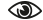der Tisch (7.1.1) = tableder Löffel (7.3) = spoonder Student (7.1.2) = studentder Pullover (7.3) = pulloverdie Tüte (7.1.2) = plastic bagdas Bett (7.1.2) = bedder Garten (7.2.3) = gardendie Pflanze (7.1.2) = plantder Schrank (7.2.1) = closetder Nagel (7.2.3) = nailder Finger (7.3) = fingerder Idiot (7.1.2) = idiotdas Bein (7.1.1) = legder Brief (7.1.1) = letterdas Dach (7.2.2) = roofder Laden (7.2.3) = shopdie Karotte (7.3) = carrotdie Diskussion (7.1.2) = discussiondas Haar (7.1.1) = hairder Schlüssel (7.3) = keydie Straße (7.1.2) = streetdie Stadt (7.2.1) = towndie Wolke (7.1.2) = cloudder Vorhang (7.2.1) = curtainder Turm (7.2.1) = towerdie Uhr (7.1.2) = clockcontact privacy statement imprint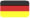GERMAN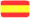SPANISH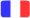FRENCH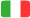ITALIAN